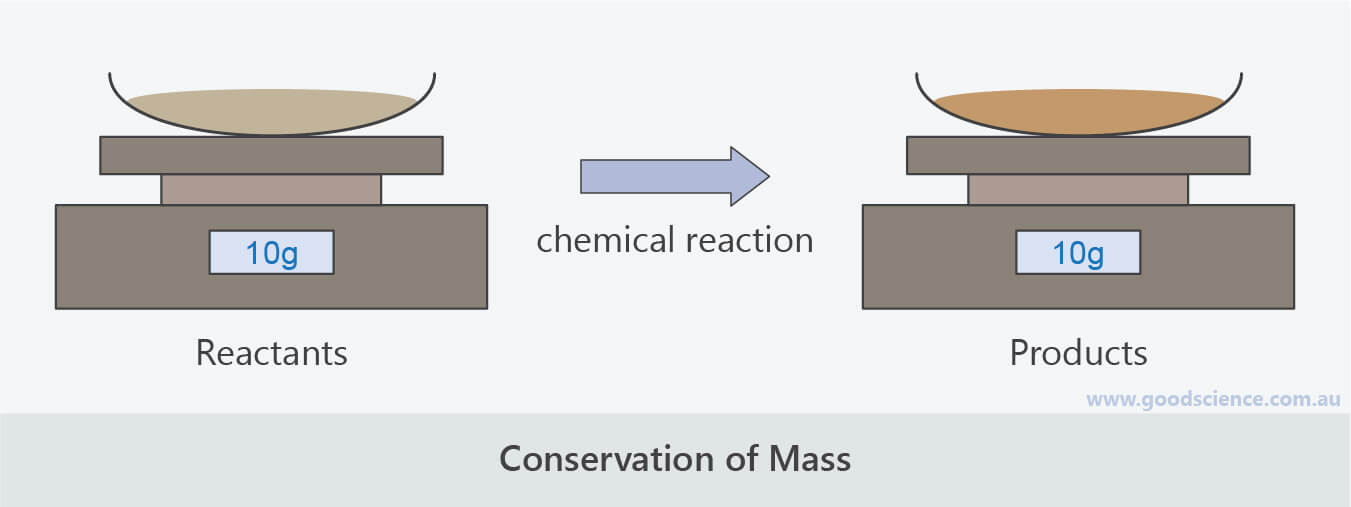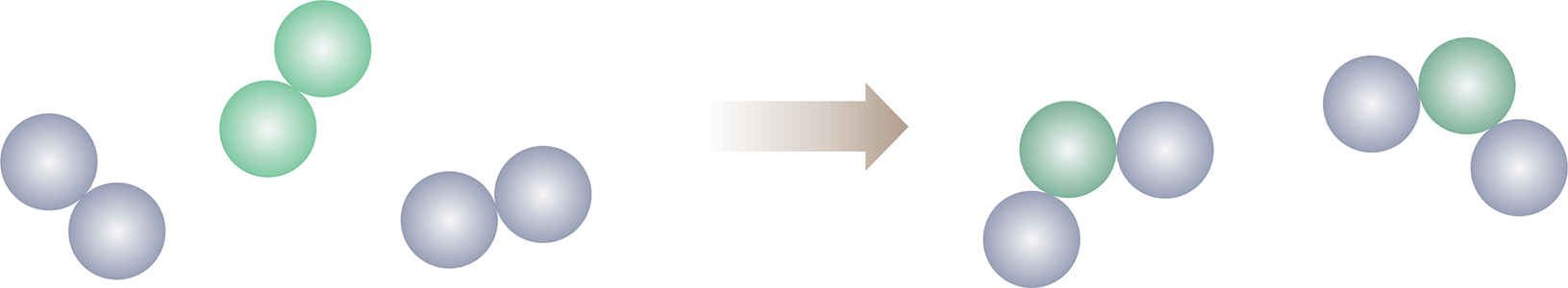Learning Objective

In this lesson we will revise how to balance a chemical equation to reflect the conservation of mass during a chemical reaction.

Learning Outcomes

By the end of this lesson you will be able to:

• Write word equations and formula equations to represent chemical reactions.
• Include states of matter in formula equations.
• Balance formula equations to reflect conservation of mass.

Balancing Chemical Equations

1 | Describing Chemical Reactions With Equations

2 | Conservation of Mass

3 | Steps for Balancing a Chemical Equation

4 | Tips for Balancing Chemical Equations

5 | Summary

Click images to preview the worksheet for this lesson and the Year 10 Chemistry Workbook (PDF and print versions)

## Describing Chemical Reactions With Equations

• A chemical reaction involves one or more substances, known as reactants, interacting to form one or more new substances, known as products.
• A chemical equation is a short-hand format that represents a chemical reaction.
• The general format of a chemical equation is:• A chemical equation can be written as a word equation or a formula equation.
• A word equation contains the chemical names of reactants and products.
• Example
• calcium hydroxide  +  carbon dioxide    calcium carbonate  +  water
• A formula equation contains the chemical formulas of reactants and products.
• Example
• Ca(OH)2  +  CO2    CaCO3  +  H2O
• Formula equations may also include the states of matter of reactants and products.
• These are written in brackets after each chemical formula:
• (s) represents solids.
• (l) represents liquids.
• (g) represents gases.
• (aq) represents aqueous solutions, which are substances that are dissolved in water, such as acids and soluble salts.
• Example
• Ca(OH)2 (aq)  +  CO2 (g)    CaCO3 (s)  +  H2O (l)

## Conservation of Mass

• The Law of Conservation of Mass states that matter is neither created nor destroyed.
• This means that even though chemical reactions result in the formation of new substances, the total mass of reactants equals the total mass of products.
• In other words, the total numbers of each type of atom are the same before and after a chemical reaction has occurred – they are just arranged differently.
• Consider the previous example:
• Ca(OH)2  +  CO2    CaCO3  +  H2O
• There is 1 calcium atom on each side of the equation.
• There are 4 oxygen atoms on each side of the equation.
• There are 2 hydrogen atoms on each side of the equation.
• Therefore, this equation shows the conservation of mass.
• However, it is not usually the case that a formula equation will show the conservation of mass.
• Consider the following equation:
• FeCl3  +  K2S    Fe2S3  +  KCl
• There is 1 iron atom on the left side of the equation and 2 iron atoms on the right side of the equation.
• There are 3 chlorine atoms on the left side of the equation and 1 chlorine atom on the right side of the equation.
• There are 2 potassium atoms on the left side of the equation and 1 potassium atom on the right side of the equation.
• There is 1 sulfur atom on the left side of the equation and 3 sulfur atoms on the right side of the equation.
• Therefore, this equation does not show the conservation of mass.
• This doesn’t mean that the reaction can’t take place, it simply means that the reactants and products do not occur in a 1:1:1:1 ratio as they do in the previous example.
• The equation is said to be unbalanced.
• For the equation to be balanced, the ratios of substances in the equation need to be adjusted so there are equal numbers of each type of atom on both sides of the equation.
• This is done by placing coefficients (numbers) in front of the chemical formulas of substances.The mass of the products of a chemical reaction is equal to the mass of the reactants.

## Steps for Balancing a Chemical Equation

• Balancing equations successfully requires a systematic approach.
• We will illustrate this using the previous reaction between iron (III) chloride and potassium sulfide as an example.

Step 1

• Write the formula equation showing all reactants and products.
• FeCl3  +  K2S    Fe2S3  +  KCl

Step 2

• Tally up the total numbers of each type of atom for both sides of the equation.
 Atom Reactants Products Fe 1 2 Cl 3 1 K 2 1 S 1 3

Step 3

• Place coefficients in front of substances until there are equal numbers of each type of atom on both sides of the equation.
• Each time a coefficient is added, update the tally.
• Placing a ‘2’ in front of FeCl3 will balance the number of Fe atoms:
• 2 FeCl3  +  K2S    Fe2S3  +  KCl
• It will also change the number of Cl atoms on the left side.
• The updated tally is:
 Atom Reactants Products Fe 2 2 Cl 6 1 K 2 1 S 1 3
• The iron atoms are now balanced, but the other atoms are still unbalanced.
• Placing a ‘6’ in front of KCl will balance the number of Cl atoms:
• 2 FeCl3  +  K2S    Fe2S3  +  6 KCl
• It will also change the number of K atoms on the right side.
• The updated tally is:
 Atom Reactants Products Fe 2 2 Cl 6 6 K 2 6 S 1 3
• The iron and potassium atoms are now balanced, but the other atoms are still unbalanced.
• Placing a ‘3’ in front of K2S will balance the number of K atoms as well as the number of S atoms:
• 2 FeCl3  +  3 K2S    Fe2S3  +  6 KCl
• The updated tally is:
 Atom Reactants Products Fe 2 2 Cl 6 6 K 6 6 S 3 3
• The equation is now balanced.
• It shows that, for this reaction, the ratio of substances is 2:3:1:6.
• (There is no need to place a ‘1’ in front of substances in a balanced equation.)

## Tips for Balancing Chemical Equations

• Only adjust one coefficient at a time.
• Remember that coefficients change the tallies for all atoms in a substance, not just the atom you are trying to balance.
• If the equation contains elements, leave them until last when adjusting coefficients. This is because they can be adjusted without affecting the tallies of other atoms.
• You can only balance an equation by placing coefficients in front of substances. Never adjust the subscript numbers that are part of a chemical formula.
• For example, you can’t change H2O to H2O2, it must be written as 2 H2O if you wish to double the number of oxygen atoms.
• Only place whole numbers (2, 3, 4 etc) in front of chemical formulas. If you find that a fraction is required to balance an equation, multiply all coefficients by the smallest number required to convert the fraction to a whole number.
• Polyatomic groups, such as NO3 and SO4, often stay together in reactions. If this is the case, consider them as one group in the tally, rather than as individual atoms – this will simplify the process.
• Check that the coefficients are the smallest possible numbers.
• For example,  4 H2 + 2 O2 4 H2O  can be simplified to  2 H2 + O2 2 H2O.If your equation contains an element, balance it last.

## Summary

• A word equation shows the chemical names of all reactants and products involved in a chemical reaction.
• A formula equation shows the chemical formulas of reactants and products.
• Formula equations may also include the states of matter of reactants and products, in brackets after each chemical formula.
• (s) represents solids.
• (l) represents liquids.
• (g) represents gases.
• (aq) represents aqueous solutions.
• A balanced equation shows the ratios of reactants and products.
• A balanced equation reflects the Law of Conservation of Mass and shows that atoms are neither created nor destroyed during a chemical reaction, but only rearranged.
• Steps for balancing a chemical equation:
• Write the formula equation showing all reactants and products.
• Tally up the total numbers of each type of atom for both sides of the equation.
• Place coefficients in front of reactants and products until there are equal numbers of each type of atom on both sides of the equation.